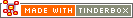^contains( target, data )^Code Type: Boolean Comparison Code Scope of Action: item

^contains( target, data )^

If the data (string or pattern) is somewhere within the target string this condition is true. Comparisons are always case-sensitive.

If the data is a pattern this means a fixed string is substituted with a regular expression; it may contain wild cards, character ranges, etc.

examples:

`^contains(Lemon Tree,emo)^` is: true

`^contains(Lemon Tree,ora)^` is: false

`^contains(^text^, blah)^` (search this note's body text)

`^contains(^title(parent)^, blah)^` (do as above but for its parent)

`^contains(^directory^, blah)^` (check this note's path)

`^contains(^text^, [0-9])^` (search this note's body text for numerical characters)

Note that the beginning of line marker character, ^ (as used in Regular Expression patterns) needs to be doubled because Tinderbox would otherwise interpret it as beginning a mark-up element. Thus:

`^contains(^text,^^P)^` looks for lines beginning with a capital P

 Up: Boolean Comparison Previous: ^beginsWith( target, data )^ Next: ^days( date, N )^

[Last updated: 14 Dec 2009, using v5.0]